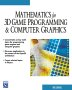# Maths - Bivectors

The vector cross gives a bivector rather than a vector.

Its not very strange that the product of two vectors is not a vector itself, its the same principle as the dot product which produces a scalar. In fact the cross product and dot products are complimentary.

In 3 dimensions a bivector behaves like a vector therefore, in most cases, we treat the result of cross product as a vector.

However there are some advantages in keeping track as to whether such quantities are vectors or bivectors:

• In physics, it can give us clues about the nature of a quantity, bivectors represent quantities associated with planes including rotations.
• There are some small differences between the algebra of bivectors, the dot product of two bivectors is a negative number whereas the dot product of two vectors is a positive number.
• This allows the vector cross product to be generalised to the outer product which is defined for all dimensions of vectors.
• This is a good introduction to Clifford Algebra/Geometric Algebra which is a more general type of algebra and gives a lot of insights

Multiplying the bivector by a vector will toggle it back to a vector, so it is like an exclusive-or operation, multiplying the same types gives a bivector multiplying different types gives a vector:

vector × vector = bivector
bivector × bivector = bivector
vector × bivector = vector
bivector × vector = vector

## Vector Cross Product

For a more general introduction to bivectors see the pages about Clifford Algebra/Geometric Algebra.

 metadata block see also: tutorial Correspondence about this page Book Shop - Further reading. Where I can, I have put links to Amazon for books that are relevant to the subject, click on the appropriate country flag to get more details of the book or to buy it from them.Mathematics for 3D game Programming - Includes introduction to Vectors, Matrices, Transforms and Trigonometry. (But no euler angles or quaternions). Also includes ray tracing and some linear & rotational physics also collision detection (but not collision response).Covers VRML 2 (but not the upcoming X3D standard). A good introduction to all VRML2 node types and how to build them. Other Math Books Specific to this page here:

This site may have errors. Don't use for critical systems.